Math & Science Home | Proficiency Tests | Mathematical Thinking in Physics | Aeronauts 2000

 CONTENTS Introduction Fermi's Piano Tuner Problem How Old is Old? If the Terrestrial Poles were to Melt... Sunlight Exerts Pressure Falling Eastward What if an Asteroid Hit the Earth Using a Jeep to Estimate the Energy in Gasoline How do Police Radars really work? How "Fast" is the Speed of Light? How Long is a Light Year? How Big is a Trillion? "Seeing" the Earth, Moon, and Sun to Scale Of Stars and Drops of Water If I Were to Build a Model of the Cosmos... A Number Trick Designing a High Altitude Balloon Pressure in the Vicinity of a Lunar Astronaut Space Suit due to Outgassing of Coolant Water Calendar Calculations Telling Time by the Stars - Sidereal Time Fields, an Heuristic Approach The Irrationality ofThe Irrationality ofThe Number (i)i Estimating the Temperature of a Flat Plate in Low Earth Orbit Proving that (p)1/n is Irrational when p is a Prime and n>1 The Transcendentality ofIdeal Gases under Constant Volume, Constant Pressure, Constant Temperature and Adiabatic Conditions Maxwell's Equations: The Vector and Scalar Potentials A Possible Scalar Term Describing Energy Density in the Gravitational Field A Proposed Relativistic, Thermodynamic Four-Vector Motivational Argument for the Expression-eix=cosx+isinx Another Motivational Argument for the Expression-eix=cosx+isinx Calculating the Energy from Sunlight over a 12 hour period Calculating the Energy from Sunlight over actual full day Perfect Numbers-A Case Study Gravitation Inside a Uniform Hollow Sphere Further note on Gravitation Inside a Uniform Hollow Sphere Pythagorean Triples Black Holes and Point Set Topology Additional Notes on Black Holes and Point Set Topology Field Equations and Equations of Motion (General Relativity) The observer in modern physics A Note on the Centrifugal and Coriolis Accelerations as Pseudo Accelerations - PDF File On Expansion of the Universe - PDF File

## Estimating the Temperature of a Flat Plate in Low Earth Orbit

This problem is a real-world problem that I first encountered in my daily work at NASA. A flat plate is orbiting the Earth at a mean altitude of 300 km. Its orbital velocity is 7.5 x 103 m/sec eastward. (All spacecraft are launched eastward to take advantage of the Earth's rotational motion.) The plate is in sunlight. Sunlight warms the plate, and the plate radiates thermal energy back into space.

Ambient atomic and ionic species are also present. These species are moving at characteristic thermal velocities, which are very small compared to the plate's orbital velocity and may, for all intents and purposes, be ignored. The flat plate may be thought of as 'running into' populations of stationary particles at a velocity of 7.5 x 103 m/sec. From the plate's point of view, these particle populations appear to be ramming it 'head-on' at 7.5 x 103 m/sec. When the particles hit the plate, they are assumed to loose their kinetic energy to the plate as heat. Again, as in the case for sunlight, the plate also radiates this energy back into space.

The balance between incoming energy and outgoing (radiated) energy causes the plate to come to an equilibrium temperature. The problem is to calculate this temperature.

We begin with Stefan-Boltzmann's law for a black body in sunlight.

SSUN =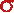T4 W/m2

This law enables us to estimate the temperature T of such an object, assuming that we know the power per unit area SSUN falling on the plate. From direct measurement, we know that at 1 A.U,

SSUN = 1,360 W/m2.

We also know that= 5.67 x 10-8 W/(m2 K4).

Thus, the nominal temperature of an object, in space and in sunlight, is 394 °K.

Next, we must deal with the ambient particle fluxes. In general, random directional particle flux to the surface is calculated from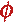=¼(nVPARTICLE) m-2-sec-1

where n is the number density of the particle species per m3, and VPARTICLE is the average velocity of the individual particles in m/s. Here, we take VPARTICLE = 7.5 x 103 m/sec. Since particle flux to the surface is assumed to be ram directional (i.e., we are assuming that the plate 'sees' all the particles coming head-on at 7.5 x 103 m/sec), the usual factor of ¼ (which arises when the directions are random) may be omitted.

From an established knowledge of the orbital environment at 300 km, we select the following, dominant particle species:

Oxygen ions: O+
Atomic oxygen: O
Molecular oxygen: O2
Molecular nitrogen: N2.

Then, for each of these selected particle species, we may define a power flux to the surface:

SPARTICLE = (nVPARTICLE)(EKINETIC/PARTICLE) W/m2.

where EKINETIC/PARTICLE is the kinetic energy per particle. The values of n and EKINETIC/PARTICLE must be determined.

If (as we have already assumed) the particles simply deliver their kinetic energy to the plate upon impact (totally inelastic collision), then they may be treated as energy fluxes additional to that of the sun, and a final temperature may be estimated from:T4 = SSUN +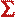SPARTICLES

Now, we are ready to calculate. We have already calculated the temperature contribution from the sun:

T = 394 °K.

Next, we calculate the kinetic energy of each of the particle species:

1. Oxygen ions: O+:

mO+ = 16 x (1.67 x 10-27 kg) = 2.67 x 10-26 kg
VO+ = 7.5 x 103 m/sec
EK/O+ = ½ mO+ (VO+)2 = 7.51 x 10-19 j

2. Atomic oxygen: O:

mO = 16 x (1.67 x 10-27 kg) = 2.67 x 10-26 kg
VO = 7.5 x 103 m/sec
EK/O = ½ mO (VO)2 = 7.51 x 10-19 j

3. Molecular oxygen: O2:

mO2 = 32 x (1.67 x 10-27 kg) = 5.34 x 10-26 kg
VO2 = 7.5 x 103 m/sec
EK/O2 = ½ mO2 (VO2)2 = 1.50 x 10-18 j

4. Molecular nitrogen: N2:

mN2 = 28 x (1.67 x 10-27 kg) = 4.68 x 10-26 kg
VN2 = 7.5 x 103 m/sec
EK/N2 = ½ mN2 (VN2)2 = 1.32 x 10-18 j

The number densities of each of the particle species at the altitude of 300 km are obtained from published references (cited):

nO+ = 5 x 1011 /m3 (Sp. & Planetary Env., Vol. 1, pg. 2-31)
nO = 2 x 1014 /m3 (U.S. Std. Atm., pg. 30)
nO2 = 1011 /m3 (U.S. Std. Atm., pg. 30)
nN2 = 5 x 1012 /m3 (U.S. Std. Atm., pg. 30)

Using these quantities, we obtain the power fluxes S for each of the particle species:

SO+ = 2.82 x 10-3 W/m2
SO = 1.13 W/m2
SO2 = 1.12 x 10-3 W/m2
SN2 = 4.96 x 10-2 W/m2

For convenience, we sum these contributions:SPARTICLES = SO+ + SO + SO2 + SN2 = 1.18 W/m2.

Recall that SSUN = 1,360 W/m2, so that the temperature contribution from the ram particles alone must be very small in comparison. Let us estimate this ram particle temperature contribution.

An estimation of the change in temperature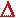T due toSPARTICLES may be obtained from10SPARTICLES = 4T3T

With T = 394 °K, andSPARTICLES = 1.18 W/m2, we findT = 8.51 X 10-2 K. This value is too small to make a significant difference when compared to the contribution of the sun. We therefore ignore it, and take the plate's temperature to be 394 °K.

10 To obtain this expression, let us re-write our working equations as follows: Set S = SSUN, and set S +S = SSUN +SPARTICLES . Then, set S =T4 and S +S =(T +T)4 =(T4 + 4T3T + 6T2(T)2 + 4T(T)3 + (T)4)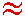(T4 + 4T3T) forT<<T. Subtracting the two expressions and substittingS =SPARTICLES gives the expression in the text.